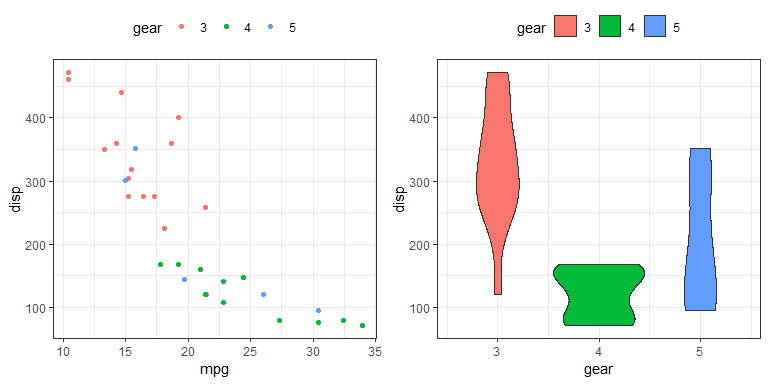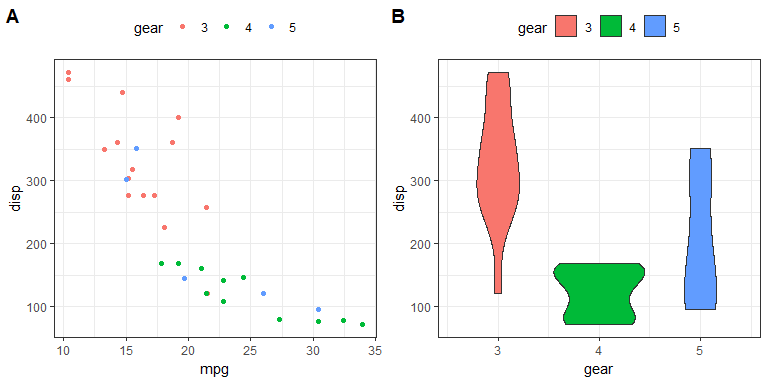# How to combine plots and table made with ggplot (or grid graphics) in R

October 1, 2018
By

[This article was first published on Roman Luštrik not Inc. - R, and kindly contributed to R-bloggers]. (You can report issue about the content on this page here)
Want to share your content on R-bloggers? click here if you have a blog, or here if you don't.

In the last few years, a number of options of how to combine grid graphics (incl. `ggplot2`) have emerged.
If you can’t remember all functions to do this, this post may serve as a reference guide, but it’s mostly
for me because I keep forgetting the functionalities provided by `cowplot` and `patchwork` packages (thank you Jaap). Here appearing in order I encountered them.

```library(ggplot2)

# prepare some figures
fig1 <- ggplot(mtcars, aes(x = mpg, y = disp, color = as.factor(gear))) +
theme_bw() +
theme(legend.position = "top") +
scale_color_discrete(name = "gear") +
geom_point()

fig2 <- ggplot(mtcars, aes(x = gear, y = disp, fill = as.factor(gear))) +
theme_bw() +
theme(legend.position = "top") +
scale_fill_discrete(name = "gear") +
geom_violin()
```

## gridExtra

Here is oldie but goldie from Baptiste’s `gridExtra` package.
You can, for example, specify the layout matrix or specify number of columns. The below code chunk is using `ggplot2::ggsave` which saves the last subplot only, which is why I saved the result of `grid.arrange` into a new variable (but see `cowplot` below).

```library(gridExtra)

fig.combined1 <- grid.arrange(fig1, fig2, ncol = 2)
ggsave(plot = fig.combined1, filename = "grid.arrange.png",
width = 8, height = 4, units = "in", dpi = 96)
```## cowplot

Next is `cowplot`, which comes with nice vignettes. What I like about this package is the elegant adding of letters to subplots, e.g.

```library(cowplot)

plot_grid(fig1, fig2, labels = "AUTO")
cowplot::ggsave(filename = "plotgrid.png", width = 8,
height = 4, units = "in", dpi = 96)
```

See the vignettes for more information. Package also packs its own `ggplot` which masks the function `ggplot2::ggsave` (read: `ggsave` from package `ggplot2`). The beauty of it is that it saves the gridded plot, not the last (sub)plot.## patchwork

Thomas did a great job of making combining of plots trivially easy. The GitHub repository of the package is a rich source of information and I could never do it justice. Here’s my botched attempt of just an example of what the package functionality provides.

```library(patchwork)

fig1 + fig2
cowplot
```R-bloggers.com offers daily e-mail updates about R news and tutorials about learning R and many other topics. Click here if you're looking to post or find an R/data-science job.
Want to share your content on R-bloggers? click here if you have a blog, or here if you don't.